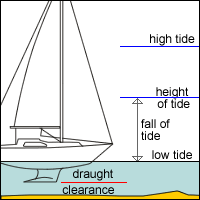Help for
Sailors and
Yachtsmen

Find the mimimum depth to anchor and how much anchor line

Question: Given the following data, work out the minimum depth of water to anchor in and how much anchor line to let out, allowing for a scope of 3:1.

Present height of tide: 4.0m 4.2m 2.4m 1.0m 1.0m

 Minimum depth: Anchorline (rounded up):

Explanation:To find the minimum depth, you need to find a depth which, at low tide, will equal the draught and clearance. To find this:

1. subtract the next low water from the present height of tide to get the fall of tide. (if you are staying for more than one low water, choose the lowest).
2. add the draught and clearance to this fall to find the minimum depth to anchor.

To find the amount of anchor line to let out, there are two formulas to find the depth at high water:

high water depth = high tide – height of tide + mooring depth (as worked out above)
or
high water depth = high tide – low tide + draught + clearance

This is the depth of water at high tide. Multiply by the required scope to find the length of cable/line to deploy.